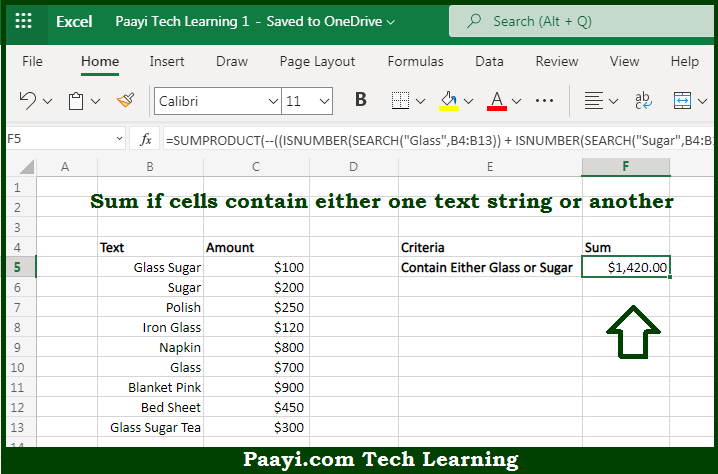# Learn How to SUM If Cells Contain Either x or y in Microsoft Excel

Written by | 0 Comments | 579 Views

In this article, you will learn how to SUM various things in Microsoft Excel using a single/combination(s) of functions. You will also know how to SUM If Cells Contain Either x or y and see the generic formula.

SUM If Cells Contain Either x or y in Microsoft Excel

The main purpose of this formula is to sum if cells contain either one text string or another. Here we will learn how to sum if cells is having either x or y in the workbook in Microsoft Excel. That implies, with the help of a formula based on the SUMPRODUCT function you can able to sum if cells contain either one text string or another. So, with the help of this formula, you can able to sum if cells is having either x or y in the workbook in Microsoft Excel.

General Formula to SUM If Cells Contain Either x or y

=SUMPRODUCT(--((ISNUMBER(SEARCH("cat",rng1))+ ISNUMBER(SEARCH("rat",rng1)))>0),rng2)

The Explanation for the SUM If Cells Contain Either x or ySo we know that with the help of the given formula above you can able to sum if cells contain either one text string or another. Here we will learn how to sum if cells is having either x or y in the workbook in Microsoft Excel. As we know that the while we do sum cells with "OR" criteria, we should be careful, not double count when there is a possibility that both criteria will return true. In the provided example, we need to sum values in the targeted Column. We can't use SUMIFs with two criteria, because SUMIFS is based on AND logic. And if we try to use two SUMIFS, that is  "SUMIFS + SUMIFS" we will double count because there are cells that contain both. So, with the help of this formula, you can able to sum if cells is having either x or y in the workbook in Microsoft Excel.